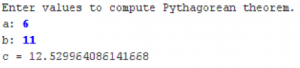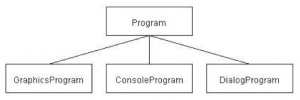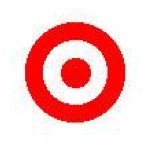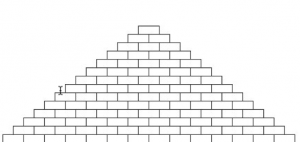## cs106a – Assignment #2 – Task #4

In high-school geometry, you learned the Pythagorean theorem for the relationship of the lengths of the three sides of a right triangle:
a2 + b2 = c2
which can alternatively be written as:
c = √(a2 + b2)
Most of this expression contains simple operators covered in Chapter 3. The one piece that’s missing is taking square roots, which you can do by calling the standard function Math.sqrt. For example, the statement

`double y = Math.sqrt(x);`

sets y to the square root of x.
Write a ConsoleProgram that accepts values for a and b as ints and then calculates the solution of c as a double. Your program should be able to duplicate the following sample run:cs106a – assignment #2 – task #4

## cs106a – Assignment #2 – Task #3

Write a GraphicsProgram subclass that draws a partial diagram of the acm.program class hierarchy, as follows:cs106a – assignment #2 – task #3
The only classes you need to create this picture are GRect, GLabel, and GLine. The major part of the problem is specifying the coordinates so that the different elements of the picture are aligned properly. The aspects of the alignment for which you are responsible are:

• The width and height of the class boxes should be specified as named constants so that they are easy to change.
• The labels should be centered in their boxes. You can find the width of a label by calling label.getWidth() and the height it extends above the baseline by calling label.getAscent(). If you want to center a label, you need to shift its origin by half of these distances in each direction.
• The connecting lines should start and end at the center of the appropriate edge of the box.
• The entire figure should be centered in the window.

## cs106a – Assignment #2 – Task #2

Suppose that you’ve been hired to produce a program that draws an image of an archery target—or, if you prefer commercial applications, a logo for a national department store chain—that looks like this:cs106a – assignment #2 – task #2
This figure is simply three GOval objects, two red and one white, drawn in the correct order. The outer circle should have a radius of one inch (72 pixels), the white circle has a radius of 0.65 inches, and the inner red circle has a radius of 0.3 inches. The figure should be centered in the window of a GraphicsProgram subclass.

To draw a circle create an oval with using the diameter (twice the radius), and place it at the given position:
Continue reading “cs106a – Assignment #2 – Task #2”

## cs106a – Assignment #2 – Task #1

Write a GraphicsProgram subclass that draws a pyramid consisting of bricks arranged in horizontal rows, so that the number of bricks in each row decreases by one as you move up the pyramid, as shown in the following sample run:cs106a – assignment #2 – task #1
The pyramid should be centered at the bottom of the window and should use constants for the following parameters:

```   BRICK_WIDTH    The width of each brick (30 pixels)
BRICK_HEIGHT   The height of each brick (12 pixels)
BRICKS_IN_BASE The number of bricks in the base (14)
```

The numbers in parentheses show the values for this diagram, but you must be able to change those values in your program.

## Assignment #2 Extra Task #1

Please note, this blog entry is from a previous course. You might want to check out the current one.

Enhance your application to show the user error conditions like divide by zero, square root of a negative number, and insufficient operands. One way to do this (and to get more experience with using id) might be to have runProgram:usingVariableValues: in CalculatorBrain return an id (instead of a double) which is an NSNumber with the result or an NSString with the description of an error if it encounters one. Then update your Controller to use introspection on the return value and display the appropriate thing to the end-user.

Mainly the API of the CalculatorBrain has to be changed from double to id:
Continue reading “Assignment #2 Extra Task #1”